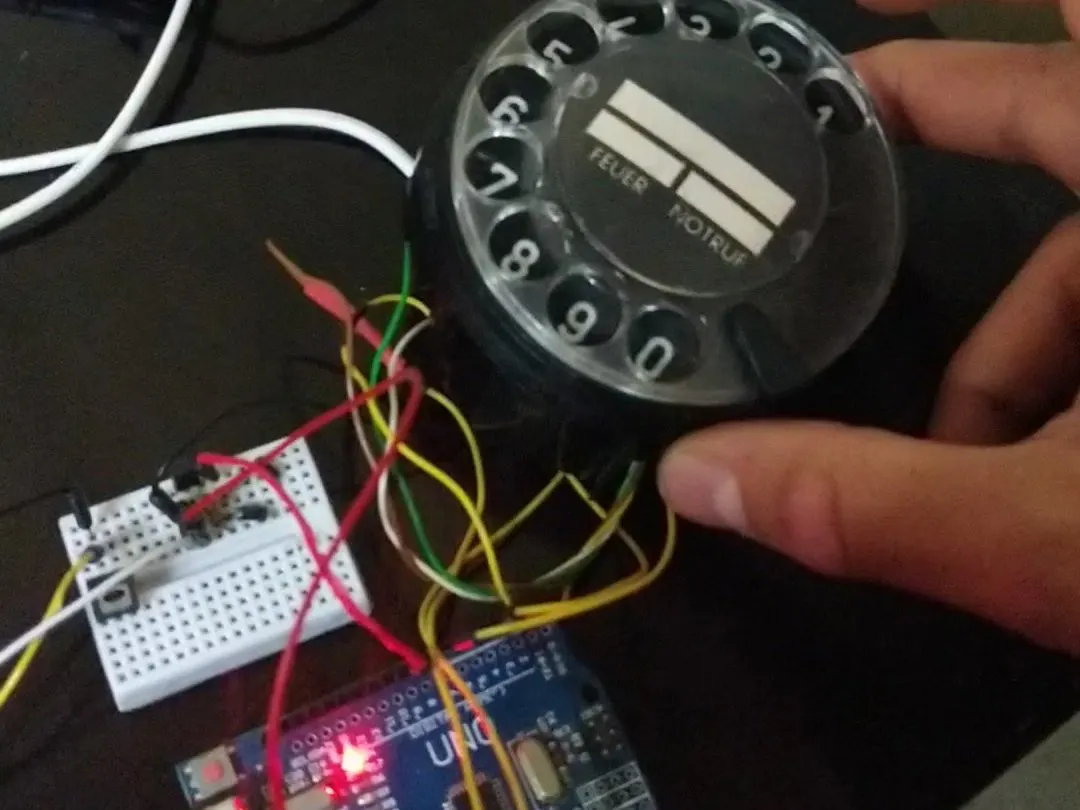Project tutorialLet the old phone disk function in some thing new using the Gear motor to open the door

• 2 views
• 0 respects

## Apps and online services

### What is the project.

Rotary Dial Disk (Rotary Phone) Password programmed by Arduino Uno.

A 6 Digits Password To Open The Door By A Gear Motor. If The Password Is Wrong The LED Will Blink Sever Times. After Putting The Write Password You Can Power Anything But In This App. A Door Lock Opens.

### Why making it.

To let the kids use the old rotary phone to do something new.

The RDD has less wires than the original keypad.

### The easy of the code.

The Code has been Written In Very Simple Way No-one had Done Yet. This Is The Only Code That Applies The RDD In This Way Of Coding.

Easy Code. I Used The Basic Commands To Write The Code.

The Number Of Digits Can Be Changed.

All The Code Has been Written Without Any Timers.

No Libraries Used In The Code.

### How does it work.

The RDD Has Four Wires As In (Figure 1) Two for Pulses For One Digit Entered (the upper two conductors). And Two As Switch (the lower two conductors) That Close After One Digit's End.

The Four Wires of the RDD Connected To The Yellow Wires As In (Figure 2).as Pulse Pin To Digital Pin 3 The Other To GND Ground. Signal Pin To Digital Pin 7 The Other To 5V Source. The Two Red Wires To The

### How Does The Code Work.

The Code Is Counting The Pulses Saving It To The First Digit. Then To The Second Digit. And So on.the number of digits makes the password. each password could be entered to do sever things as you program.

## Code

##### The Code Of The ProjectArduino
```  //Rotary Dial Disk

//By Aws AlKarmi   //By Aws AlKarmi   //By Aws AlKarmi   //By Aws AlKarmi   //By Aws AlKarmi   //By Aws AlKarmi
// [[[[[[[[[[[[[[[[[[[[[[[[[[[[[[[[[[[[[[[[[[[[[[[[[[[[[[[[[[[[[[[[[[[[[[[[[[[[[[[[[[[[[[[[[[[[[[[[[[[[[[[[[[[[[

// Four Wires Out Of It ...... Two For Pulse Counting .... And .... Two For Ending The Digit 'Pulses'

#define pulsePin 3         //Pulse Pin To Digital Pin 3 The Other To GND Ground
int number;             //Useing Array Way To Record The Number
#define signal1 7          //Signal Pin To Digital Pin 7 The Other To 5V Source
int currentDigit=0;         //Initializing By 0
int pulseCount=0;           //Initializing By 0
#define led LED_BUILTIN        //Working With The LED Built In
int state=1;                  //Initializing State
int d=70;                    // The Delay Time For LED To Blink At The Wrong Password
int state2=0;               //Initializing State2
#define motor1 8           //The Main Pin To Work With
#define motor2 10         //The AUX Pin To Work With

void setup()
{

Serial.begin(9600);              //Begining The Serial
pinMode(pulsePin,INPUT);         //pulsePin As INPUT
pinMode(signal1,INPUT);          //signal   As INPUT
pinMode(led,OUTPUT);             //led      As OUTPUT
pinMode(motor1,OUTPUT);          //motor1   As OUTPUT
pinMode(motor2,OUTPUT);          //motor2   As OUTPUT

digitalWrite(led,LOW);       //Initializing First Command 'not necessary'
digitalWrite(motor1,LOW);    //Initializing First Command 'not necessary'
digitalWrite(motor2,HIGH);   //Initializing First Command 'not necessary'

}
void loop(){                     //The Main Program

if (digitalRead(signal1)==1&&state==1)    // Putting State 'Not Necessary'

{
if (digitalRead(pulsePin)!=0)         // If The Circuit Opened

{
delay(80);            // Debouncing Time 'Can Be Adjusted'
pulseCount++;         // Count The Pulses
Serial.println(pulseCount);   // Print On Serial Monitor Expected Number From 1 To 10
}
}
else
if(digitalRead(signal1)!=1&&pulseCount>0)              // If Signal Not Equal 1 'The Pulses Stoped'
{                                                // AND Pulse Count Is More Than 0 'A Digit Entered'

if (currentDigit<6)    //If The Number Of Digits Not Equal The Full Number Of Digits Then
{
Serial.print("  The Entered Digit .... ");         //Print On Serial Monitor
Serial.println(pulseCount);                       //Print On Serial Monitor

number[currentDigit]= pulseCount;                   //Recording The Digit Entered
Serial.print("The Number Of Digits Entered Yet .... ");   //Print On Serial Monitor
Serial.println(currentDigit+1);                       //Print On Serial Monitor
Serial.print("The Digits Entered Yet .... ");             //Print On Serial Monitor The Password
for(int i=0;i<7;i++)
{
Serial.print(number[i]);
}

Serial.println(" ...... By Aws Karmi ......");         // Print On Serial Monitor My Name
currentDigit++;                      // The Next Digit To Record On
pulseCount=0;                        // Reset The Pulses Counting
number[currentDigit]=0;              // Initializing The Next Digit By 0
state2=1;                            // Putting State To 1
}
if (currentDigit==6)                  //If we Exeed 6 Digits
{
if (number==4&&number==3&&number==4&&number==3&&number==2&&number==2) //If The Digits Equal
Serial.println(" ///////WRITE Answer//////");          //Print On Serial Monitor
digitalWrite(motor1,HIGH);             //Open The Door
digitalWrite(motor2,LOW);

digitalWrite(led,HIGH);
delay(1000);                            // LED AND Motor1 Work For 1 Second Delay
digitalWrite(motor1,LOW);
digitalWrite(motor2,HIGH);

digitalWrite(led,LOW);
delay(500);
digitalWrite(led,HIGH);
delay(500);
digitalWrite(led,LOW);

for (int i=0;i<7;i++)                           // Reset The Numbers 'Password'
{
number[i]=0;
}

state2=2;                                    //Putting State To 2
currentDigit=0;                              //Reset The Number Of Digits

}else if(state2==1)                             // Checking The State Condition
{
for (int i=0;i<7;i++)                          // Reset The Numbers 'Password'
{
number[i]=0;
}

Serial.println(" ///////WRONG Answer//////");                //Print On Serial Monitor

for(int j=0;j<15;j++)                          // Blinking The LED
{
digitalWrite(led,HIGH);
delay(d);
digitalWrite(led,LOW);
delay(d);
}

currentDigit=0;                                     //Reset The Number Of Digits

}

}

}

```

#### Arduino and Android Based Password Protected Door Lock

Project tutorial by Md. Khairul Alam

• 19,617 views
• 60 respects

#### Arduino as a Voltmeter

Project tutorial by AJ-Explains-It-All

• 2,926 views
• 6 respects

#### Android App-Based Home Automation System Using IOT

Project tutorial by Team Autoshack

• 52,735 views
• 148 respects

#### rfid attendance system using arduino with GSM

Project tutorial by sarful

• 21,601 views
• 31 respects

#### Smart Door with Face Unlock

Project tutorial by Divins Mathew

• 16,280 views